# Rhombus

"What is a rhombus?" asked Miss Granger in geometry class.

Nicole, who had always loved geometry said, "A rhombus is also a parallelogram, but because of its unique properties, it gets an individual identity as a quadrilateral."

When you ask someone the question: 'What is a rhombus?,' then Nicole's answer is almost perfect.

In this section, we’re going to look at the rhombus definition, understand the rhombus shape, learn about the area of a rhombus, as well as its perimeter. But before we get into those details, let's also look at properties of a rhombus, and its unique diamond geometric shape.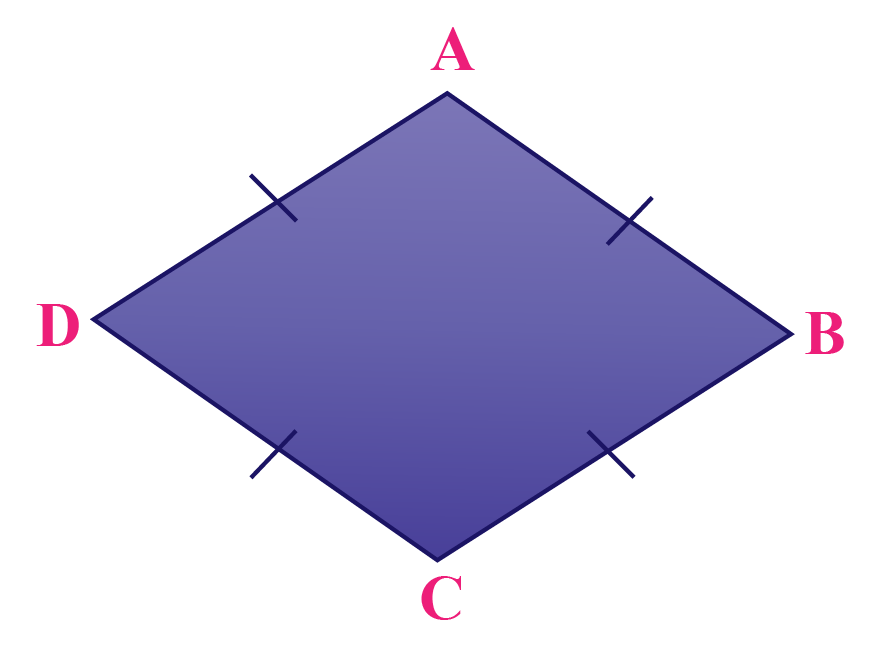## Lesson Plan

 1 What is a Rhombus? 2 Important Notes on Rhombus 3 Solved Examples on Rhombus 4 Interactive Questions on Rhombus 5 Tips and Tricks

## What is a Rhombus?

The rhombus is an interesting quadrilateral because of its unique shape.

While all its sides are equal, it is not a square because all its angles are $$90^\circ$$.

In this chapter, we will explain the properties of a rhombus and why it's quite a cool quadrilateral.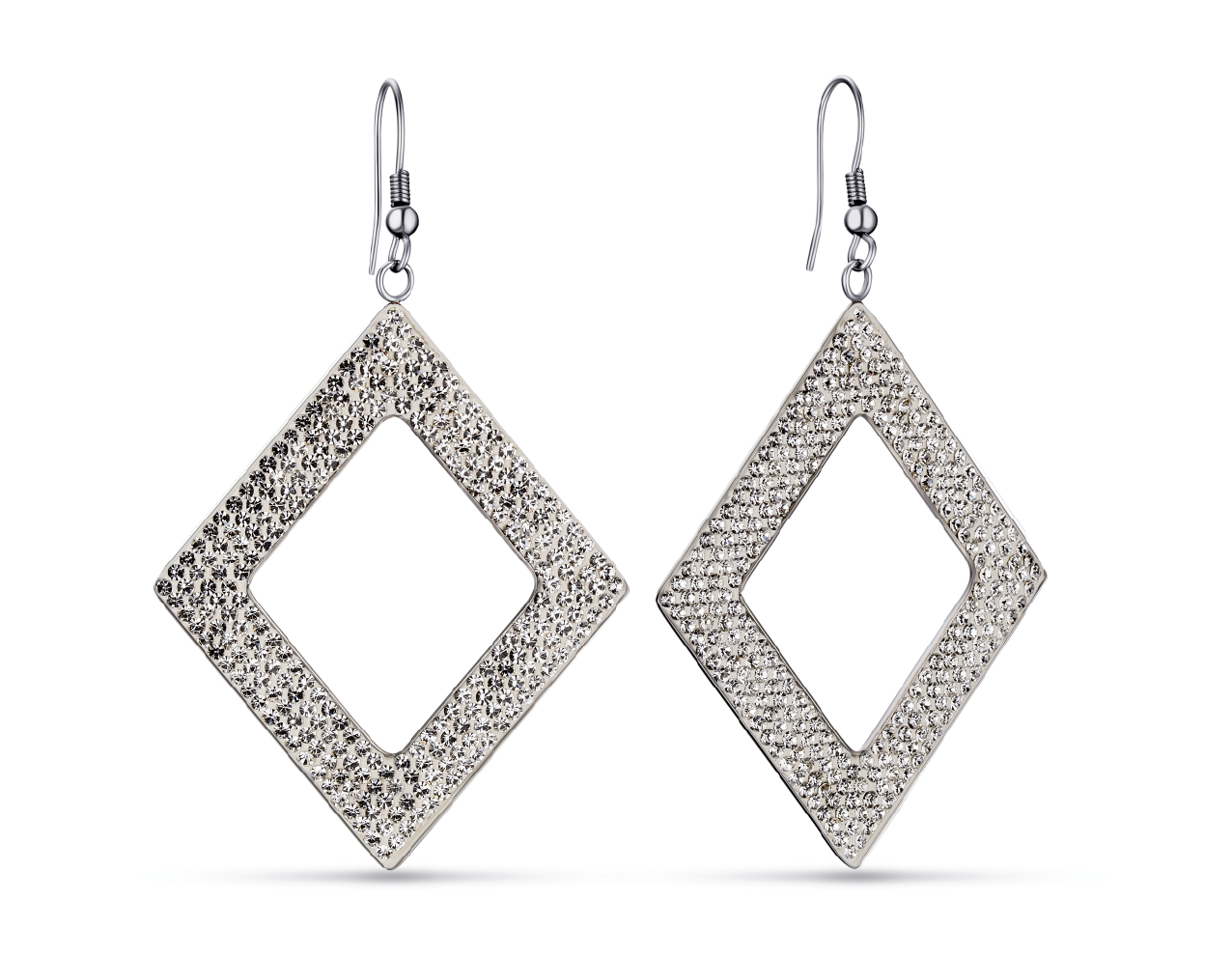Like most things in math, the name "rhombus" comes from Ancient Greece from the word "rhombos," which means a piece of wood whirled on a string to make a roaring noise. Today, however, we see rhombus-shaped figures every day. Look at the diamond in a baseball field or those cool earrings you spotted at the mall the other day.

Rhombus definition: A rhombus can be defined as a diamond-shaped quadrilateral that has all four sides equal. But isn’t that a square? Yes, as a matter of fact, it is.

But how is a rhombus different? Well, unlike a square where all sides and angles are equal, the rhombus shape may show that it has all four sides equal, but only its opposite angles are equal.

## Properties of a Rhombus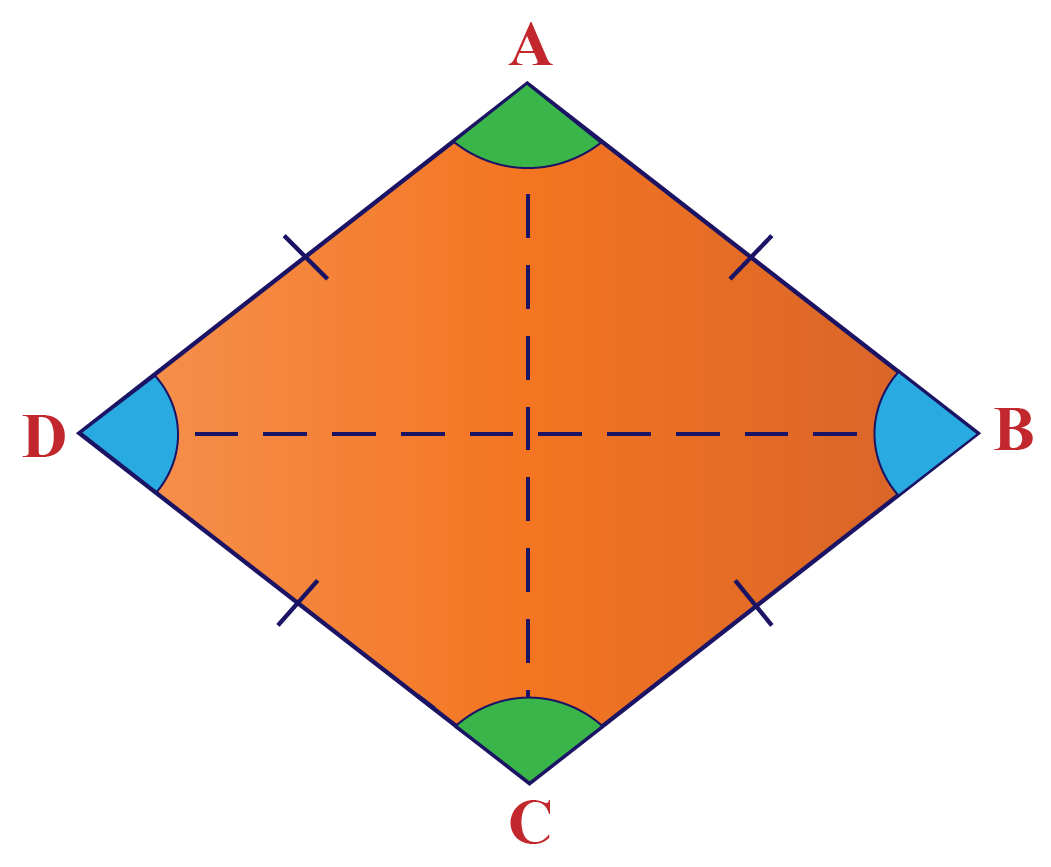A rhombus, like all geometric shapes, has properties that are unique to it. These include:

• All four sides of a rhombus are equal.
• Opposite sides of a rhombus are parallel.
• Opposite angles of a rhombus are equal.
• Diagonals bisect each other at 90° or right angles.
• The sum of two adjacent angles is 180°.
• All rhombuses are parallelograms, but all parallelograms are not rhombuses.
• All rhombuses are not squares, but all squares are rhombuses.

## Formulae for a Rhombus

The formulae for rhombuses are defined for two features:

1. Area of a rhombus, where $$A = \dfrac{{d_1 d_2 }}{2}$$
2. Perimeter of a rhombus, where $$P = 4\times \text{side}$$

## Area and Perimeter of a Rhombus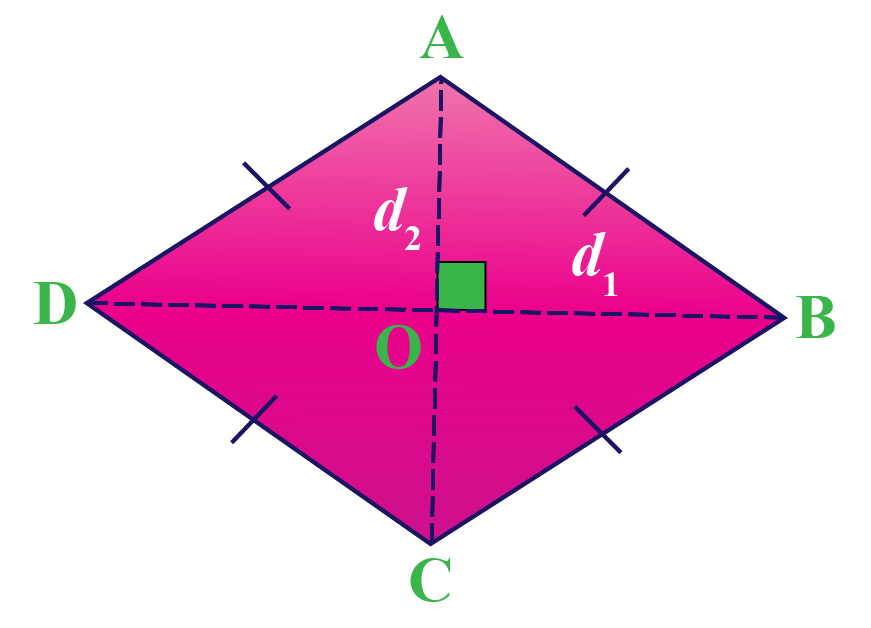### Area of a Rhombus

The area of a rhombus is defined as a product of the two diagonals divided by 2

 $$A = \dfrac{{d_1 d_2 }}{2}$$

Where $$d_1$$ and $$d_2$$ are the lengths of the diagonals.

### Perimeter of a RhombusSince all four sides of a rhombus are equal, much like a square, the formula for the perimeter is the product of the length of one side with 4

 $$P = 4 \times \text{side}$$

## Angles of a Rhombus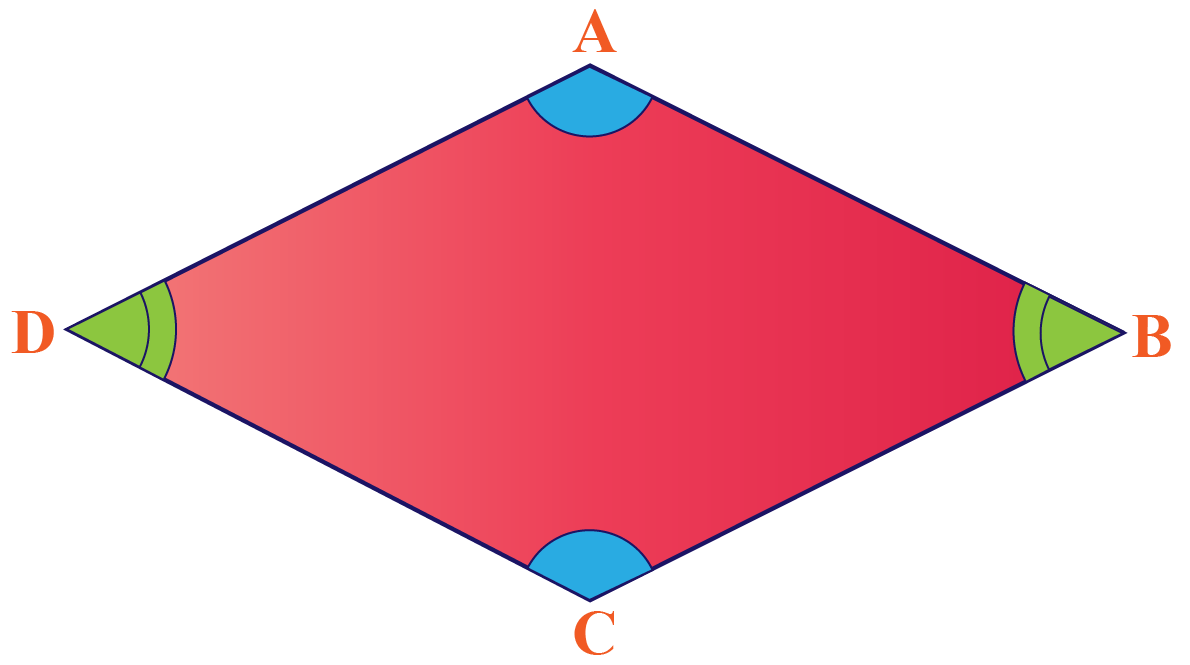As mentioned in the properties of a rhombus, the sum of two adjacent angles is 180°.

So if one of the angles is 60°, then the adjacent angle will be 120°.

Also, opposite angles of a rhombus are equal, which means summing up the four angles will give you 360°, i.e. the number of degrees that can be measured in any quadrilateral.

## Diagonals of a Rhombus

One thing you should remember about the diagonal of a rhombus is that it always bisects at a 90° angle, which means that the two sides bisected will be the same length.

For example: if the length of a diagonal is 10 cm and the other diagonal bisects it, then it is divided into two 5 cm diagonals.

Unless the rhombus is a square, then the diagonals will have different values.

If you know the side of the rhombus and the value of certain angles, then you can determine the length of the diagonal.

More Important Topics
Numbers
Algebra
Geometry
Measurement
Money
Data
Trigonometry
Calculus